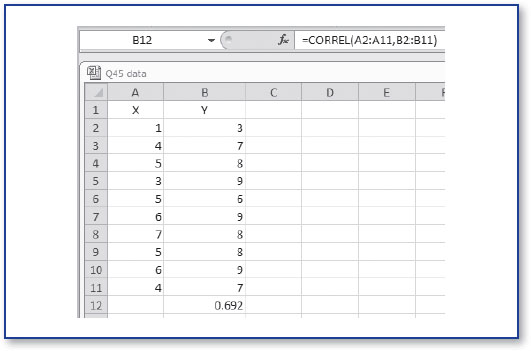In an increasingly data-driven world, it is more important than ever for students as well as professionals to better understand basic statistical concepts. 100 Questions (and Answers) About Statistics addresses the essential questions that students ask about statistics in a concise and accessible way. It is perfect for instructors, students, and practitioners as a supplement to more comprehensive materials, or as a desk reference with quick answers to the most frequently asked questions.

# How Can I Use Excel to Compute a Correlation Coefficient?

### How Can I Use Excel to Compute a Correlation Coefficient?

To compute the correlation coefficient using Excel, you use the =CORREL function. We will be using the same set of data you saw in question #44.

If you are using a Mac or a PC, follow these steps:

• Enter =CORREL in the cell where you want the results to appear.
• Enter the first data array, a comma, and then the second data array. The completed function should appear as shown in Figure 45.1.
• Press the Enter key, and as you will see, the correlation of .69 (rounded to the nearest 100th) is returned to the cell in which the function is located.

### Figure 45.1 The =CORREL functionIf you are using the Windows version of Excel, you can also ...

•••